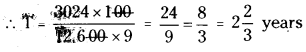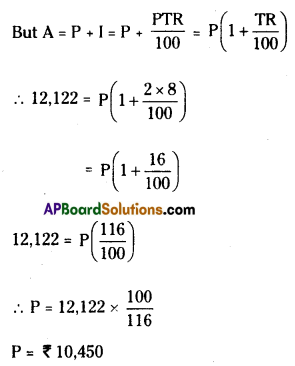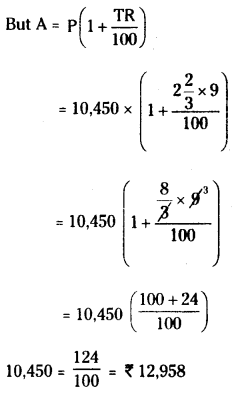# HSSlive: Plus One & Plus Two Notes & Solutions for Kerala State Board

## AP Board Class 7 Maths Chapter 6 Ratio Applications Ex 6 Textbook Solutions PDF: Download Andhra Pradesh Board STD 7th Maths Chapter 6 Ratio Applications Ex 6 Book AnswersAP Board Class 7 Maths Chapter 6 Ratio Applications Ex 6 Textbook Solutions PDF: Download Andhra Pradesh Board STD 7th Maths Chapter 6 Ratio Applications Ex 6 Book Answers

## Andhra Pradesh State Board Class 7th Maths Chapter 6 Ratio Applications Ex 6 Books Solutions

 Board AP Board Materials Textbook Solutions/Guide Format DOC/PDF Class 7th Subject Maths Chapters Maths Chapter 6 Ratio Applications Ex 6 Provider Hsslive

2. Click on the Andhra Pradesh Board Class 7th Maths Chapter 6 Ratio Applications Ex 6 Answers.
3. Look for your Andhra Pradesh Board STD 7th Maths Chapter 6 Ratio Applications Ex 6 Textbooks PDF.
4. Now download or read the Andhra Pradesh Board Class 7th Maths Chapter 6 Ratio Applications Ex 6 Textbook Solutions for PDF Free.

## AP Board Class 7th Maths Chapter 6 Ratio Applications Ex 6 Textbooks Solutions with Answer PDF Download

Find below the list of all AP Board Class 7th Maths Chapter 6 Ratio Applications Ex 6 Textbook Solutions for PDF’s for you to download and prepare for the upcoming exams:

Question 1.
How long will it take for a sum of ₹ 12600 invested at 9% per annum beocme to ₹ 15624?
Solution:
HereA = ₹ 15,624 R = 9% T = ?
P = ₹ 12,600
∴ I = A – P = 15,624 – 12,600 = ₹ 3024
Also I = 𝑃𝑅𝑇100
∴ 3024 = 12,600×9×T100Question 2.
At what rate a sum doubles itself in 8 year 4 months?
Solution:
Given that A = double the sum
Let the principle be P
then A = 2P
T =8 years 4 months = 8y 412 y = 813 years = 253
R = R% say
We know that
I =  PTR 100
Here I = A – P
= 2P – P = P
∴ P = 𝑃⋅253×𝑅100
∴ R = 100 x 325 = 12%

Question 3.
A child friendly bank announces a savings scheme for school children. They will give kiddy banks to children. Children have to keep their savings in it and the bank collects all the money once in a year. To encourage children savings, they give 6% interest if the amount exceeds by ₹ 10000. and other wise 5%. Find the interest received by a school if they deposit is ₹ 9000 for one year.
Solution:
Money deposited = ₹ 9000
Interest applicable 5% on ₹ 9000
= 5 × 9000100 = ₹ 450

Question 4.
A sum of money invested at 8% per annum for simple interest amounts to ₹ 12122 in 2 years. What will it amounts to in 2 year 8 months at 9% rate of interest?
Solution:
First part
Pirnciple = P say
R=8%
T = 2 years
A = ₹ 12,122Second part
P = ₹10,450
R = 9%
T = 2 years 8 months, A = ?Question 5.
In 4 years, ₹ 6500 amounts to ₹ 8840 at a certain rate of interest. In what time will ₹ 1600 amounts to 1816 at the same rate?
Solution:
First part
T = 4 years
P = ₹ 6500
A = ₹ 8840
R = R% = ?
I = A – P = 8840 – 6500 = ₹ 2340
But I = 𝑃𝑇𝑅100
∴ 2340 = 6500×4×𝑅100
∴ R = 2340×10𝜃6500×4=364 = 9%

Second part
P = ₹ 1600
A = ₹ 1816
T = ?
R = 9%
I = A – P = 1816 – 1600 = ₹ 216
But I = 𝑃𝑇𝑅100
∴ 2340 = 1600×𝑇×9100
∴ R = 216×100𝜃1600×9=32 = 1 12 years.

## Andhra Pradesh Board Class 7th Maths Chapter 6 Ratio Applications Ex 6 Textbooks for Exam Preparations

Andhra Pradesh Board Class 7th Maths Chapter 6 Ratio Applications Ex 6 Textbook Solutions can be of great help in your Andhra Pradesh Board Class 7th Maths Chapter 6 Ratio Applications Ex 6 exam preparation. The AP Board STD 7th Maths Chapter 6 Ratio Applications Ex 6 Textbooks study material, used with the English medium textbooks, can help you complete the entire Class 7th Maths Chapter 6 Ratio Applications Ex 6 Books State Board syllabus with maximum efficiency.

## FAQs Regarding Andhra Pradesh Board Class 7th Maths Chapter 6 Ratio Applications Ex 6 Textbook Solutions

#### Can we get a Andhra Pradesh State Board Book PDF for all Classes?

Yes you can get Andhra Pradesh Board Text Book PDF for all classes using the links provided in the above article.

## Important Terms

Andhra Pradesh Board Class 7th Maths Chapter 6 Ratio Applications Ex 6, AP Board Class 7th Maths Chapter 6 Ratio Applications Ex 6 Textbooks, Andhra Pradesh State Board Class 7th Maths Chapter 6 Ratio Applications Ex 6, Andhra Pradesh State Board Class 7th Maths Chapter 6 Ratio Applications Ex 6 Textbook solutions, AP Board Class 7th Maths Chapter 6 Ratio Applications Ex 6 Textbooks Solutions, Andhra Pradesh Board STD 7th Maths Chapter 6 Ratio Applications Ex 6, AP Board STD 7th Maths Chapter 6 Ratio Applications Ex 6 Textbooks, Andhra Pradesh State Board STD 7th Maths Chapter 6 Ratio Applications Ex 6, Andhra Pradesh State Board STD 7th Maths Chapter 6 Ratio Applications Ex 6 Textbook solutions, AP Board STD 7th Maths Chapter 6 Ratio Applications Ex 6 Textbooks Solutions,
Share: